GROUP THEORY SYMBOL

# GTSGGetIreps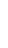GTSGGetIreps[space group, k-point, reciprocal basis]
gives the character table and the representation matrices of a space group at the wave vector k

## DetailsDetails

• The algorithm is based on an induction scheme as proposed by Aroyo et al.
•  GOVerbose True Controls if output is printed GOGeneralPositionIreps True Controls if the character table and representation matrices of the full space group or the character table of the space group of k is printed.
• M. I. Aroyo et al., Acta Crystallographica Section A, A62, 115128 (2006).
• W. Hergert, M. Geilhufe, Group Theory in Solid State Physics and Photonics. Problem Solving with Mathematica, chapter 6.

## ExamplesExamplesopen allclose all

### Basic Examples  (1)Basic Examples  (1)

• First load the package.
•  In:=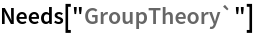• We choose the monoclinic space group P21/c (#14). We first specify the monoclinic basis vectors and calculate the corresponding basis vectors of the reciprocal lattice using GTReciprocalBasis. Then we install the space groups using GTInstallGroup.
•  In:=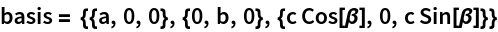Out=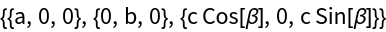In:=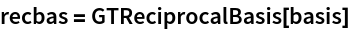Out=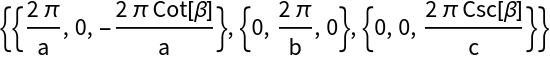In:=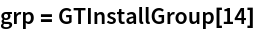Out=In:=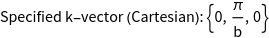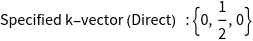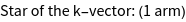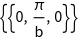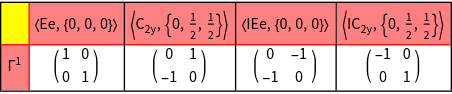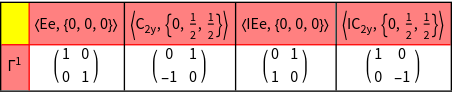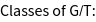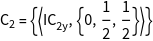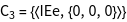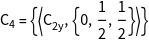Out=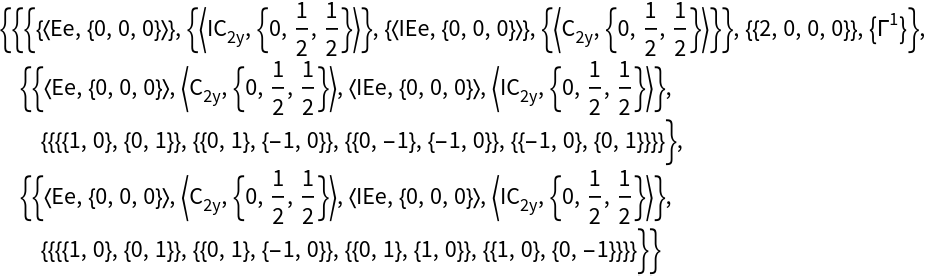## See AlsoSee Also

GTSGCharacterTableGTGetIrep